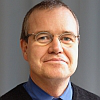# Methods and Algorithms of Numerical Simulation## 课程介绍

Numerical analysis is playing a key role in the design and development of technical products in all branches of mechanical engineering and automotive development. The extensive use of various kinds of computer-aided engineering tools has become indispensable for product development. The methods used typically generate large amounts of data. These tools produce results that must be carefully analyzed and interpreted. Only in this way the benefit from using CAE in terms of quality and shortening the development can be maximized. To this end, however, a profound understanding of the underlying numerical methods and algorithms is essential. This two day course is therefore aimed at those who want to get an overview of the currently used numerical methods. The objective of the seminar is to understand the different methods and to get a sense of what is possible. The theoretical part of the course is supplemented by practical exercises on the computer with a software system for numerical analysis (MATLAB or similar).

## 谁该参加?

The seminar is designed for engineers working in mechanical engineering and vehicle development in the field of numerical simulation as well as project engineers and project managers from these areas.

## 课程内容

• Linear algebra: Basics of vector and matrix
• Direct solution of linear equations
• Solution of very large systems of equations
• Iterative solution of linear equations
• Solution of eigenvalue problems
• Numerical approximation
• Numerical Interpolation
• Numerical Integration
• Methods for solving nonlinear equations
• Solving systems of nonlinear equations
• Variational principles
• Discretization
• Numerical solution of ordinary differential equations

Prof. Dr.-Ing. Detlev Maurer
University of Applied Sciences LandshutDr. Maurer has received his PhD from the Department of lightweight design at Technical University Darmstadt led by Prof. Wissmann. Thereafter he has been working for several years in the technical analysis department of Adam Opel AG where he headed the crash simulation group. After a several years activity in Information Technology at Adam Opel AG and General Motors in Detroit (USA), he has been appointed in 2005 as a Professor at the University of Applied Sciences Landshut. He teaches mathematics, numerical methods and finite elements in the Faculty of Mechanical Engineering.

### 德国联系人Dr. Dirk Ulrich

dirk.ulrich@carhs.de

All prices are exclusive of VAT.